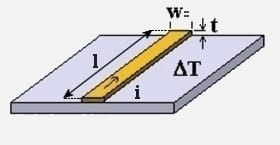Traces

## Trace Width Calculator### Inputs

Max Current
i
Trace Width Unit
w
Trace Thickness
t
Temperature Rise
ΔT

Ambient Temperature
Θ
Length
l

Trace Width:
11.8 mil
Resistance:
0.247 Ω
Voltage Drop:
0.247 Volts
Power Loss:
0.247 Watts

### Internal Trace (inner layer)

Trace Width:
30.8 mil
Resistance:
0.0951 Ω
Voltage Drop:
0.0951 Volts
Power Loss:
0.0951 Watts

View Notes

#### Calculation Notes

To ensure a trace can carry a given current capacity (I), this tool calculates the trace width required. Internal trace values are the results for a trace within a multilayer, although outer traces that are potted or insulated should also use internal values. Outer trace values are the results for a trace that is exposed to the air, normally on the top or bottom layers of a circuit.

Factors that influence the trace width calculation include:-

• Max current (i)
• Trace thickness (t)
• Max desired temperature rise (ΔT)

a_{ext} = \left( \frac{i}{0.048\times {\Delta T}^{0.44}} \right) ^\frac{1}{0.725}\; (external trace area)

w_{ext} = \frac{a_{ext}}{1.378 \times t}\; (external trace width)

a_{int} = \left( \frac{i}{0.024\times {\Delta T}^{0.44}} \right) ^\frac{1}{0.725}\; (internal trace area)

w_{int} = \frac{a_{int}}{1.378 \times t}\; (internal trace width)

References:
• Electrical Circuit Theory and Technology (ISBN 978-1-85617-770-2)
• Douglas Brooks, "Temperature Rise in PCB Traces", 1998

Disclaimer: The information and this tool are provided with no liability of any kind whatsoever, use at your own risk.